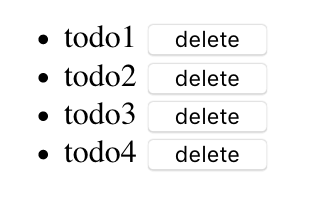Why are there two ways of declaring variables in Javascript? What is the difference between 'var' and 'let'? After reading this article you know why the let keyword has been introduced and when to use it.

# What is the difference between let and var in Javascript and when should I use one or the other?

The `let` keyword was introduced in ECMAScript 6 and adds another way to declare re-assignable variables in Javascript. The following example shows how to assign values by using let and var:

``````let a = 10;
console.log(a); // 10

var b = 20;
console.log(b); // 20
``````

When variables are declared within a function, their scope limits to that function. Both `a` and `b` have been declared outside of a function and have the same scope, which is global. This article will now focus on the differences between `var` and `let`.

## Difference 1: Window object

When we take the code from above and place it in a website, the first difference appears in the window object.

• let does not add variables to the window object
• var does add variables to the window object
``````<!DOCTYPE html>
<html lang="">
<meta charset="utf-8">
<script>
let a = 10;
console.log(window.a); // undefined

var b = 20;
console.log(window.b); // 20
</script>
<body>
</body>
</html>
``````

## Accessing values in a lexical subscope:

Since `a` and `b` are defined with var or let outside of any function, they are global variables and accessible in a lexical sub-scope:

``````let a = 10;
var b = 20;

if (true) {
console.log(a, b); // 10, 20
}
``````

## Change values in a lexical subscope:

The next example assigns two values to `a` and `b` using var. In the if-block, I re-assign `a` and re-define `b`. When the program leaves the if-block, both `a` and `b` remain their new values:

``````var a = 10;
var b = 20;

console.log(a, b); // 10, 20

if (true) {
a = 100; // re-assign
var b = 200; // re-declare within this scope
console.log(a, b); // 100, 200
}

console.log(a, b); // 100, 200
``````

Let’s do the same with let:

``````let a = 10;
let b = 20;

console.log(a, b); // 10, 20

if (true) {
a = 100; // re-assign
let b = 200; // re-declare within this scope
console.log(a, b); // 100, 200
}

console.log(a, b); // 100, 20<*=---
``````

The interesting difference is that in the line `let b = 200;` a new variable `b` is created, only accessible in the if-block. As soon as we leave the if-block, the global variable `b` is used again. I’ll let you decide if using the same variable name in different scopes is a smart thing to do. But as you see, Javascript has no problem with it.

## Accessing values in a function:

Global values defined with let or var are accessible from functions:

``````var a = 10;
let b = 20;

function test() {
console.log(a, b); // 10, 20
}

test();
``````

## Change values in a function:

The next example uses var to declare the variables `a` and `b`. In function `test()` `a` is re-assigned and `b` re-declared. The result is much like using let in our previous lexical scope example. As soon as the program returns from the function, `b` has value 20 again.

``````var a = 10;
var b = 20;

function test() {
a = 100; // re-assign
var b = 200; // re-declare within this scope
console.log(a, b); // 100, 200
}

test();
console.log(a, b); // 100, 20
``````

If we use let instead of var, the program behaves the same:

``````let a = 10;
let b = 20;

function test() {
a = 100; // re-assign
let b = 200; // re-declare within this scope
console.log(a, b); // 100, 200
}

test();
console.log(a, b); // 100, 20
``````

## Loop and anonymous functions

Imagine a list page that looks like this:Every row has a button that deletes a todo. Without actually creating buttons and click handlers, let’s simulate this by creating a list of anonymous functions. Each anonymous function in list `funcs` will print the todo ID.

``````var funcs = [];

for (var i = 0; i < 5; i++) {
funcs.push(() => console.log("delete " + i));
}

funcs.forEach(func => func());
``````

When we execute this code, the result look like this:

``````delete 5
delete 5
delete 5
delete 5
delete 5
``````

You might see why that would be a problem :-). What happens is that the anonymous function does not get values 0 to 4 but a reference to `i`, which will always be the last value from after the loop, in this case `5`. We can prevent this by not using an anonymous function or, much easier, by using let in the for-loop instead of var like this:

``````var funcs = [];

for (let i = 0; i < 5; i++) {
funcs.push(() => console.log("delete " + i));
}

funcs.forEach(func => func());
``````

Now we see the result we need:

``````delete 0
delete 1
delete 2
delete 3
delete 4
``````

## Conclusion

You’ve seen differences between let and var, like:

• whether the value is added to the window object or not
• scoping behaviour
• using loops and anonymous functions

We did not talk about whether it is a good thing to keep variables close to the scope where they are used or not. Some might argue it is better to use let so variables will be cleaned up after they are not needed anymore. I’ll let you decide what is best practice and hope this article helps you with that decision.

Written by Loek van den Ouweland on 2018-11-02.
Questions regarding this artice? You can send them to the address below.